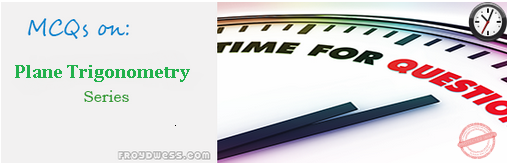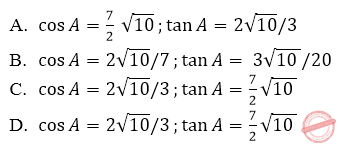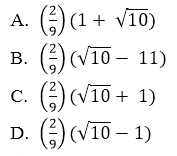# MCQ in Plane Trigonometry Part 2 | ECE Board Exam

(Last Updated On: February 5, 2020)This is the Multiple Choice Questions Part 2 of the Series in Plane Trigonometry topic in Engineering Mathematics. In Preparation for the ECE Board Exam make sure to expose yourself and familiarize in each and every questions compiled here taken from various sources including but not limited to past Board Examination Questions in Engineering Mathematics, Mathematics Books, Journals and other Mathematics References.

### Multiple Choice Questions Topic Outline

• MCQs in Trigonometry | MCQs in Solution to Right Triangles | MCQs in Pythagorean Theorem | MCQs in Solution to Oblique Triangles | MCQs in Law of Sines | MCQs in Law of Cosines | MCQs in Law of Tangents | MCQs in Trigonometric Identities | MCQs in Plane Areas (Triangles) | MCQs in Plane Areas (Quadrilaterals) | MCQs in Ptolemy’s Theorem

### Continue Practice Exam Test Questions Part II of the Series

Choose the letter of the best answer in each questions.

51. Find the supplement of an angle whose compliment is 62°.

• A. 28°
• B. 118°
• C. 152°
• D. None of these

52. A certain angle has a supplement 5 times its compliment. Find the angle.

• A. 67.5°
• B. 157.5°
• C. 168.5°­­­
• D. 186°

53. The sum of the two interior angles of the triangle is equal to the third angle and the difference of the two angles is equal to 2/3 of the third angle. Find the third angle.

• A. 15°
• B. 75°
• C. 90°
• D. 120°

54. The measure 0f 1 ½ revolutions counter-clockwise is:

• A. 540°
• B. 520°
• C. +90°
• D. -90°

55. The measure of 2.25 revolutions counterclockwise is:

• A. -835°
• B. -810°
• C. 805°
• D. 810°

56. Solve for θ: sin θ – sec θ + csc θ – tan 2θ = –0.0866

• A. 40°
• B. 41°
• C. 47°
• D. 43°

57. What are the exact values of the cosine and tangent trigonometric functions of acute angle A, given that sin A = 3/7?58. Given three angles A, B, and C whose sum is 180°. If the tan A + tan B + tan C = x, find the value of tan A x tan B x tan C.

• A. 1 – x
• B.√x
• C. x/2
• D. x

59. What is the sine of 820°?

• A. 0.984
• B. -0.866
• C. 0.866
• D. -0.500

60. csc 270° = ?

• A. -√3
• B. -1
• C. √3
• D. 1

61. If coversine Ѳ is 0.134, find the value of Ѳ.

• A. 60°
• B. 45°
• C. 30°
• D. 20°

62. Solve for cos 72° if the given relationship is cos 2A = 2 cos2  A – 1.

• A. 0.309
• B. 0.258
• C. 0.268
• D. 0.315

63. If sin 3A = cos 6B then:

• A. A + B = 180°
• B. A + 2B = 30°
• C. A – 2B = 30°
• D. A + B = 30°

64. Find the value of sin (arcos 15/17).

• A. 8/17
• B. 17/9
• C. 8/21
• D. 8/9

65. Find the value of cos [arcsin (1/3) + arctan (2/√5)]66. If sin 40° + sin 20° = sin Ѳ, find the value of Ѳ.

• A. 20°
• B. 80°
• C. 120°
• D. 60°

67. How many different value of x from 0° to 180° for the equation (2sin x – 1)(cos x + 1) = 0?

• A. 3
• B. 0
• C. 1
• D. 2

68. For what value of Ѳ (less than 2π) will the following equation be satisfied?

sin2 Ѳ + 4sinѲ + 3 = 0

• A. π
• B. π/4
• C. 3π/2
• D. π/2

69. Find the value of x in the equation csc x + cot x = 3.

• A. π/4
• B. π/3
• C. π/2
• D. π/5

70. If sec2 A  is 5/2, the quantity 1 – sin2 A is equivalent to:

• A. 2.5
• B. 0.6
• C. 1.5
• D. 0.4

71. Find sin x if 2 sin x + 3 cos x – 2 = 0.

• A. 1 & -5/13
• B. -1 & 5/13
• C. 1 & 5/13
• D. -1 & -5/13

72. If sin A = 4/5, A in quadrant II, sin B = 7/25, B in quadrant I, find sin (A + B).

• A. 3/5
• B. 2/5
• C. 3/4
• D. 4/5

73. If sin A = 2.571x, cos A = 3.06, and sin 2A = 3.939x, find the value of x.

• A. 0.350
• B. 0.250
• C. 0.100
• D. 0.150

74. If cos Ѳ = √3/2, what is the value of x if x = 1 – tan2 Ѳ.

• A. -2
• B. -1/3
• C. 4/3
• D. 2/3

75. If sin Ѳ – cos Ѳ = -1/3, what is the value of sin 2 Ѳ?

• A. 1/3
• B. 1/9
• C. 8/9
• D. 4/9

76. If x cos Ѳ + y sin Ѳ = 1 and x sin Ѳ – y cos Ѳ = 3, what is the relationship between x and y?

• A.  x2 + y2 = 20
• B. x2 – y2 = 5
• C. x2 + y2 = 16
• D. x2 + y2 = 10

77. If sin x + 1/sin x = √2  , then sin2 x + 1/sin2 x is equal to:

• A. √2
• B. 1
• C. 2
• D. 0

78. The equation 2 sin Ѳ + 2 cos Ѳ – 1 = √3 is:

• A. An identity
• B. A parametric equation
• C. A conditional equation
• D. A quadratic equation

79. If x + y = 90°, then ((sin x tan y)/(sin y tan x))  is equal to:

• A. tan x
• B. cos x
• C. cot x
• D. sin x

80. if cos Ѳ = x / 2 then 1 – tan2 Ѳ  is equal to:

• A. (2×2 + 4)/x2
• B. (4 – 2×2)/x2
• C. (2×2 – 4)/x
• D. (2×2 – 4)/x2

81. Find the value in degrees of arcos (tan 24°).

• A. 61.48
• B. 62.35
• C. 63.56
• D. 60.84

82. arctan [2 cos ((√3)/2)] is equal to:

• A. π/3
• B. π/4
• C. π/6
• D. π/2

83. Solve for x in the equation: arctan (2x) + arctan (x) = π/4

• A. 0.821
• B. 0.218
• C. 0.281
• D. 0.182

84. Solve for x from the given trigonometric equation:

arctan (1 – x) + arctan (1 + x) = arctan 1/8

• A. 4
• B. 6
• C. 8
• D. 2

85. Solve for y if y = (1/sin x – 1/tan x)(1 + cos x)

• A. sin x
• B. cos x
• C. tan x
• D. sec2 x

86. Solve for x: x = (tan θ + cot θ)2 sin2 θ – tan 2 θ.

• A. sin θ
• B. cos θ
• C. 1
• D. 2

87. Solve for x: x = 1 – (sin θ – cos θ)2

• A. sin θ cos θ
• B. -2 cos θ
• C. cos 2θ
• D. sin 2θ

88. Simplify cos4 θ – sin4 θ

• A. 2
• B. 1
• C. 2 sin2 θ + 1
• D. 2 cos2 θ – 1

89. Solve for x: x = ((1 – tan2 a)/(1 + tan2 a))

• A. cos a
• B. sin 2a
• C. cos 2a
• D. sin a

90. which of the following is different from the others?

• A. 2 cos 2x – 1
• B. cos 4x – sin 4x
• C. cos 3x – sin 3x
• D. 1 – 2 sin 2x

91. Find the value of y: y = (1 + cos 2θ) tan θ.

• A. cos θ
• B. sin θ
• C. sin 2θ
• D. cos 2θ

92. The equation 2 sinh x cosh x is equal to:

• A. ex
• B.e-x
• C. sinh 2x
• D. cosh 2x

93. Simplifying the equation sin2 θ(1 + cot2 θ)

• A. 1
• B. sin2 θ
• C. sin2 θ sec2 θ
• D. sin2 θ

94. If tan θ = x2, which of the following is incorrect?

• A. sin θ = 1/√(1 + x4)
• B. sec θ = √(1 + x4)
• C. cos θ = 1/√(1 + x4)
• D. csc θ = √(1 + x4) / x2

95. In an isosceles right triangle, the hypotenuse is how much longer than its sides?

• A. 2 times
• B. √2 times
• C. 1.5 times
• D. None of these

96. Find the angle in mils subtended by a line 10 yards long at a distance of 5000 yards.

• A. 2.5 mils
• B. 2 mils
• C. 4 mils
• D. 1 mil

97. The angle or inclination of ascend of a road having 8.25% grade is _____degrees.

• A. 5.12 degrees
• B. 4.72 degrees
• C. 1.86 degrees
• D. 4.27 degrees

98. The sides of a right triangle is in arithmetic progression whose common difference if 6 cm. its area is:

• A. 216 cm2
• B. 270 cm2
• C. 360 cm2
• D. 144 cm2

### Online Questions and Answers in Plane Trigonometry Series

Following is the list of multiple choice questions in this brand new series:

Plane Trigonometry MCQs
PART 1: MCQs from Number 1 – 50                        Answer key: PART I
PART 2: MCQs from Number 51 – 100                   Answer key: PART II
PART 3: MCQs from Number 101 – 150                   Answer key: PART III

Please do Subscribe on YouTube!

P inoyBIX educates thousands of reviewers and students a day in preparation for their board examinations. Also provides professionals with materials for their lectures and practice exams. Help me go forward with the same spirit.

“Will you subscribe today via YOUTUBE?”

Subscribe